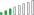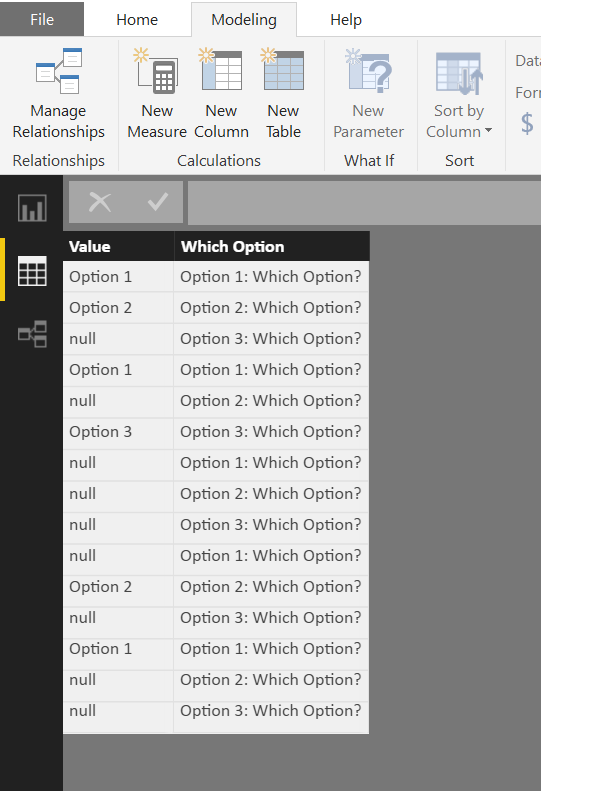cancel
Showing results for
Did you mean:New Member

## Visualization of a multiple choices question in a bar chart

Hi,

I would like to create a bar chart but havent found an easy way yet to do so. I have no problem when all the data is within one column , but sometime I need to summarize a question with data in multiple column.

My data has one column for each possible answer. As below :I

I need to build one bar chart graph summarizing the results of the data for "Which Option?" that would look like thatI have tried many things but fail as my data is structured that way.

Can someone help ?

1 ACCEPTED SOLUTIONCommunity Champion

You can do this with both DAX and Power Query

With DAX....... Go to Modelling Tab and press the NEW TABLE button...

```NEW TABLE =
VAR TotalRows =
COUNTROWS ( TableName )
RETURN
UNION (
ROW (
"Option", "Option 1",
"Count", DIVIDE (
CALCULATE (
COUNTROWS ( TableName ),
TableName[Option 1: Which Option?] = "Option 1"
),
TotalRows
)
),
ROW (
"Option", "Option 2",
"Count", DIVIDE (
CALCULATE (
COUNTROWS ( TableName ),
TableName[Option 2: Which Option?] = "Option 2"
),
TotalRows
)
),
ROW (
"Option", "Option 3",
"Count", DIVIDE (
CALCULATE (
COUNTROWS ( TableName ),
TableName[Option 3: Which Option?] = "Option 3"
),
TotalRows
)
)
)```Regards
Zubair

4 REPLIES 4Resolver III

Hello,

For this issue i recommend creating a measure for each option ( as long as it's about three in your real data aswell )
Use the COUNT() function to count the times option 1 is used in column 1 + the times option 1 is used in column 2 etc...
Do this for every option and add the measures to your bar chart.

Hope this helpsCommunity Champion

You can do this with both DAX and Power Query

With DAX....... Go to Modelling Tab and press the NEW TABLE button...

```NEW TABLE =
VAR TotalRows =
COUNTROWS ( TableName )
RETURN
UNION (
ROW (
"Option", "Option 1",
"Count", DIVIDE (
CALCULATE (
COUNTROWS ( TableName ),
TableName[Option 1: Which Option?] = "Option 1"
),
TotalRows
)
),
ROW (
"Option", "Option 2",
"Count", DIVIDE (
CALCULATE (
COUNTROWS ( TableName ),
TableName[Option 2: Which Option?] = "Option 2"
),
TotalRows
)
),
ROW (
"Option", "Option 3",
"Count", DIVIDE (
CALCULATE (
COUNTROWS ( TableName ),
TableName[Option 3: Which Option?] = "Option 3"
),
TotalRows
)
)
)```Regards
ZubairCommunity Champion

With Power Query, its much more convenient if you have many columns

Step # 1

Unpivot your Table by selecting all Columns. Rename the ColumnsStep # 2

Add this MEASURE to the TRANSFORMED table

```Percentage =
DIVIDE (
CALCULATE (
COUNTROWS ( TableName ),
FILTER (
ALLEXCEPT ( TableName, TableName[Which Option] ),
TableName[Value] <> "null"
)
),
CALCULATE (
COUNTROWS ( TableName ),
ALLEXCEPT ( TableName, TableName[Which Option] )
)
)```

Regards
ZubairFrequent Visitor

Hi,

Above approach of creating a seperate table will work one way, i.e. if the slicer/filters are from master data, data in new table can be sliced accordingly, whereas if we click on newly created response (from new table), it will not bring any change in charts created from master data.

Can that be resolved.

Regards,

Parth